Courses

# Test: MCQs (One or More Correct Option): Organic Chemistry - Some Basic Principles & Technique | JEE Advanced

## 18 Questions MCQ Test Class 11 Chemistry 35 Years JEE Mains &Advance Past yr Paper | Test: MCQs (One or More Correct Option): Organic Chemistry - Some Basic Principles & Technique | JEE Advanced

Description
This mock test of Test: MCQs (One or More Correct Option): Organic Chemistry - Some Basic Principles & Technique | JEE Advanced for JEE helps you for every JEE entrance exam. This contains 18 Multiple Choice Questions for JEE Test: MCQs (One or More Correct Option): Organic Chemistry - Some Basic Principles & Technique | JEE Advanced (mcq) to study with solutions a complete question bank. The solved questions answers in this Test: MCQs (One or More Correct Option): Organic Chemistry - Some Basic Principles & Technique | JEE Advanced quiz give you a good mix of easy questions and tough questions. JEE students definitely take this Test: MCQs (One or More Correct Option): Organic Chemistry - Some Basic Principles & Technique | JEE Advanced exercise for a better result in the exam. You can find other Test: MCQs (One or More Correct Option): Organic Chemistry - Some Basic Principles & Technique | JEE Advanced extra questions, long questions & short questions for JEE on EduRev as well by searching above.
*Multiple options can be correct
QUESTION: 1

### Resonance structures of a molecule should have :

Solution:

Resonating structures differ in bonding pattern.

*Multiple options can be correct
QUESTION: 2

### Phenol is less acidic than :

Solution:

Higher the stability of the corresponding anion, more will be the acidic character of the parent compound.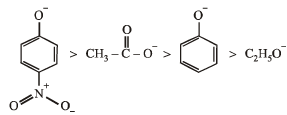Higher stability of acetate ions than phenoxide ion is due to equivalent resonating structures in the former

*Multiple options can be correct
QUESTION: 3

### Dipole moment is shown by :

Solution:

1, 4-Dichlorobenzene (p-dichlorobenzene) and trans1, 2-dichloroethene have zero dipole moment because of their symmetrical structures.

*Multiple options can be correct
QUESTION: 4

Only two isomeric monochloro derivatives are possible for:

Solution:

In n-butane, Cl can add at either the first or second carbon giving two isomers.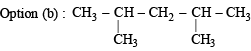will give three isomers with Cl group at either of the CH3 groups, second C-atom and 3rd C-atom.
Benzene forms only one single derivative.

Option (d) :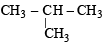will again give two isomers with Cl at either one of the CH3 groups or on the central C-atom

*Multiple options can be correct
QUESTION: 5

Which of the following have asymmetric carbon atom?

Solution:

An asymmetric carbon atom is one which is attached with 4 different groups. Hence (c) & (d) are correct.

*Multiple options can be correct
QUESTION: 6

What is the decreasing order of strength of the bases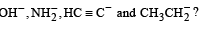Solution:

TIPS/Formulae : Conjugate base of strong acid is weak while conjugate base of a weak acid is stronger.
Acidic strength of acids, HOH > CH ≡ CH > NH3 > CH3.CH3 Hence the order of strength of bases,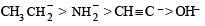*Multiple options can be correct
QUESTION: 7

Which of the following compounds will show geometrical isomerism?

Solution: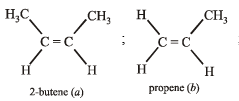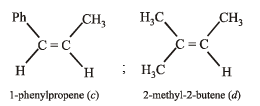Only 2-butene and 1-phenylpropene can show geometrical isomerism (cis-and trans-isomers).

*Multiple options can be correct
QUESTION: 8

Among the following compounds, the strongest acid is

Solution:

Order of acidic strength CH3OH > CH ≡ CH > C6H6 > C2H6 ; CH3OH is most acidic because O is more electronegative than C and capable of accommodating negative charge in CH3O– Although alcohols are neutral towards the litmus paper.

*Multiple options can be correct
QUESTION: 9

Tautomerism is exhibited by

Solution:

TIPS/Formulae :

For a carbonyl compound to show tautomerism, it must have at least one H at the α – carbon atom. (a), (c) and (d) show tautomerism.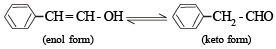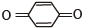Tautomerism is not possible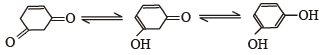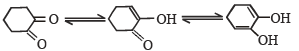*Multiple options can be correct
QUESTION: 10

An aromatic molecule will

Solution:

NOTE : An aromatic species will have :
(4n + 2) p electrons     (by Huckel’s Rule)
planar structure           (due to resonance)
cyclic structure             (due to presence of sp2 - hybrid carbon atoms).

*Multiple options can be correct
QUESTION: 11

The correct statements(s) concerning the structures E,F and G is (are) –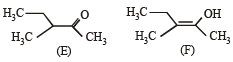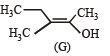Solution:

E and F ; and also E and G differ in position of atom (H), so these are tautomers (not resonating structures.
Geometrical isomers are also diastereomers).

*Multiple options can be correct
QUESTION: 12

The correct statement(s) about the compound given below is (are)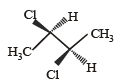Solution:

The given molecule although posseses neither centre of symmetry nor a plane of symmetry (hence optically active) but it has an axis of symmetry (Cn).
NOTE : A Cn axis of symmetry is an axis about which the molecule can be rotated by 360°/n to produce a molecule indistinguishable from the original molecule.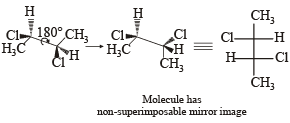*Multiple options can be correct
QUESTION: 13

The correct statement(s) about the compound

H3C(HO)HC–CH = CH – CH(OH)CH3 (X) is(are)

Solution: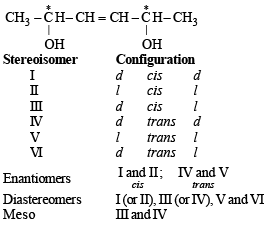*Multiple options can be correct
QUESTION: 14

In the Newman projection for 2,2-dimethylbutane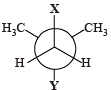X and Y can respectively be

Solution:

Structural formula of 2, 2-dimethylbutane is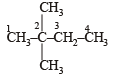(I) Newman projection using C1–C2 bond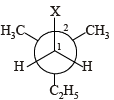(II) Newman projection using C3–C2 bond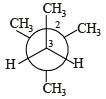*Multiple options can be correct
QUESTION: 15

Amongst the given options, the compound(s) in which all the atoms are in one plane in all the possible conformations (if any), is (are)

Solution:
*Multiple options can be correct
QUESTION: 16

Which of the following molecules, in pure form, is (are) unstable at room temperature ?

Solution:

b and c, being antiaromatic, are unstable at room temperature.

*Multiple options can be correct
QUESTION: 17

Which of the given statement(s) about N, O, P and Q with respect to M is (are) correct ?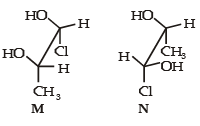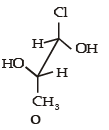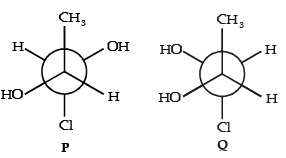Solution:

Converting all the structures in the Fischer projection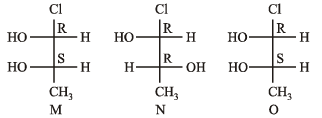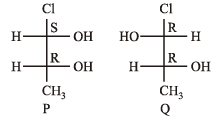M and N are diastereoisomers M and O are identical M and P are enantiomers M and Q are diastereoisomers Hence, the correct options are a, b, c.

*Multiple options can be correct
QUESTION: 18

The hyperconjugative stabilities of tert-butyl cation and 2-butene, respectively, are due to

Solution: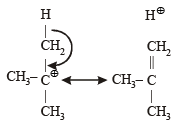In tert butyl cation, carbon bearing positive charge has one vacant p-orbital hence it is σ–p (empty) conjugation or hyperconjugation.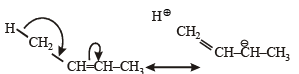In 2-butene, hyperconjugation is between σ→π* bond.